﻿ What is Current Sensor | Working Principle of Different Types of Current Sensors

# Current Sensors

### Written By:

Preeti Jain

Measuring a voltage in any system is a “passive” activity as it can be done easily at any point in the system without affecting the system performance. However, current measurement is “intrusive” as it demands insertion of some type of sensor which introduces a risk of affecting system performance.

Current measurement is of vital importance in many power and instrumentation systems. Traditionally, current sensing was primarily for circuit protection and control. However, with the advancement in technology, current sensing has emerged as a method to monitor and enhance performance.Fig. 1: A Representational Image of a Current Sensor

Knowing the amount of current being delivered to the load can be useful for wide variety of applications. Current sensing is used in wide range of electronic systems, viz., Battery life indicators and chargers, 4-20 mA systems, over-current protection and supervising circuits, current and voltage regulators, DC/DC converters, ground fault detectors, programmable current sources, linear and switch-mode power supplies, communications devices , automotive power electronics, motor speed controls and overload protection, etc.

CURRENT SENSING PRINCIPLES
A current sensor is a device that detects and converts current to an easily measured output voltage, which is proportional to the current through the measured path.

When a current flows through a wire or in a circuit, voltage drop occurs. Also, a magnetic field is generated surrounding the current carrying conductor. Both of these phenomena are made use of in the design of current sensors. Thus, there are two types of current sensing: direct and indirect. Direct sensing is based on Ohm’s law, while indirect sensing is based on Faraday’s and Ampere’s law.
Direct Sensing involves measuring the voltage drop associated with the current passing through passive electrical components.Fig. 2: A Diagram Explaining the Principle of Direct Sensing

Indirect Sensing involves measurement of the magnetic field surrounding a conductor through which current passes.Fig. 3: A Diagram Illustrating the Principle of Indirect Sensing

Generated magnetic field is then used to induce proportional voltage or current which is then transformed to a form suitable for measurement and/or control system.
PASSIVE ELEMENT BASED CURRENT SENSING TECHNIQUES
1.  Sense Resistors
Current sensing means developing a voltage signal which is representative of the current flowing at the particular place of interest inthe circuit. The traditional way of current sensing introduces a resistor in the path of the current to be sensed. The sense resistor can be placed in series with the inductor, switches, and the load.Thus, a current sensing resistor should be considered as a current-to-voltage converter.Fig. 4: An Image of a Current Sensing Resistor

The current sensing resistor should have following attributes
·         Low value in order to minimize power losses
Value of the current sense resistors primarily depend upon the voltage threshold of the following circuitry which is going to operate based upon the sensed current information. In circuits where amplification is available, emphasis is to minimize the voltage drop across the resistor.
Typical resistance values utilized in various control ICs are 20m? to 25m? .
·         Low inductance because of high di/dt.
Any inductance in the resistor, when exposed to high slew rate (di/dt), an inductive step voltage is superimposed upon the sense voltage and may be a cause of concern in many circuits. Hence sense resistors should have very low inductance.
·         Tight tolerance
For maximizing the current supply within the limit of acceptable current, the tolerance of the sense resistor must be ±1% or tighter.
·         Low temperature coefficient for accuracy
Normally specified in units of parts per million per degree centigrade (ppm/°C), temperature coefficient of resistance (TCR) is an important parameter for accuracy. Resistors with TCRs closer to zero, in the entire operating range should be used.
·         High peak power rating to handle short duration high current pulses.
Power rating is a driving factor for the selection of appropriate technology for sense resistors. Though the device may be intended to sense DC current, it may often experience transients.
Power derating curve provides allowable power at different temperatures.  But peak power capability is a function of energy; hence energy rating curve should be taken into account.
·         High temperature rating for reliability
Pros and Cons of current sensing resistors include:
Pros:
- Low cost
- High measurement accuracy
- Measurable current range from very low to medium
- Capability to measure DC or AC current

Cons:
- Introduces additional resistance into the measured circuit path, which may increase source output resistance and result in undesirable loading effect.
- Power loss due to power dissipation. Therefore, current sensing resistors are rarely used beyond the low and medium current sensing  applications.

## Current Sensing Techniques

2.  Current Sensing With a Copper Resistor
Instead of using a separate discrete resistor for current sensing, it is often useful to use copper trace in a printed circuit board as a low-value resistor for the purpose of current sensing. This method will have smaller power loss and also it will save on the efforts towards buying and installing a discrete resistor. But, since resistance of copper is very low, sensed voltage will also be very requiring significant amplification, or increase in the length of the resistor at the cost of PCB area. Another factor of significance is the TCR of copper (0.39 % / °C) which amounts to approx. 20 % change for 50% temperature rise.

3.  MOSFET-RDS
MOSFETs act as resistors when they are “on” and are biased in the Ohmic (nonsaturated) region. The current is determined by sensing the voltage across the drain-source of the MOSFET, if RDS of the MOSFET is known. The main drawbacks of this technique are low accuracy and switching noise from non-zero gate currents during transients, nonlinearity of RDSof the MOSFET , dependence of RDS on Cox, VT and temperature.

4.  Sense-FET Technique
This method is a practical technique used for current sensing in many new power MOSFET applications. A current-sensing FET in parallel with the power MOSFET is used. The effective width of the sense MOSFET (sense-FET) is significantly smaller (~10000 times) than the power FET. The accuracy of the sense-FET technique is about ±20%.· Sensorless (Observer) Approach
This method uses the inductor voltage to measure the inductor current. Since the voltage-current relation of the inductor is v=L*di/dt, the inductor current can be estimated by integrating the voltage over time. To avoid saturation in the integrator, it is reset periodically, and therefore only AC ripple current is estimated. The value of L also should be known for this technique.Fig. 5: A Diagram Explaining the Sense-FET Technique

5.  Average Current
This current-sensing technique uses an RC low-pass filter at the junction of the converter switches. Therefore, the voltage at filter output capacitor is the average voltage of the phase node. Consequently, the differential voltage at the input of the amplifier is the DC voltage across the inductor. VI-Average is a function of RESR (Inductor Resistance) andIL_DC (Inductor DC Current).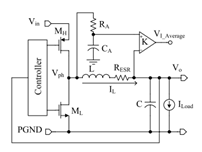Fig. 6: A Diagram Explaining the Average Current Flow

6.    Filter-Sense the inductor
This current-sensing technique uses a simple low -pass RC network to filter the voltage across the inductor and sense the current through the equivalent series resistance (ESR) of the inductor.Fig. 7: A Diagram Explaining the Filter-Sense the Inductor

## Magn. Field Based Sensing Tech.

MAGNETIC FIELD BASED CURRENT SENSING TECHNIQUES
While resistive current sensing techniques are useful in many applications, they suffer from three inherent drawbacks:
• Supply-line voltage drop
• Insertion power loss
• Common-mode errors

Most of these issues can be taken care of when sensing low to moderate amounts of current on low-voltage supply lines, but they can become significant as either currents or voltages increase. When trying to measure currents at higher levels (>10A) or where the supply line is at a high (e.g. 48V) voltage, preferred solution is to use magnetic current sensors. One of the significant and obvious benefits of using magnetic coupling for sensing current is the electrical isolation.

In these sensors, magnetically permeable core is used which concentrates the conductor’s magnetic field generated due to flow of current in the conductor.  The magnetic field is sensed using different methods:
·  Hall Effect Sensors
The Hall Effect principle states that when a current carrying conductor is placed in a magnetic field, a voltage will be generated perpendicular to the direction of the field and the flow of current.

When a constant current is passed through a thin sheet of semiconducting material, there is no potential difference at the output contacts if the magnetic field is zero. However, when a perpendicular magnetic field is present, the current flow is distorted. The uneven distribution of electron density creates a potential difference across the output terminals. This voltage is called the Hall voltage. If the input current is held constant the Hall voltage will be directly proportional to the strength of the magnetic field.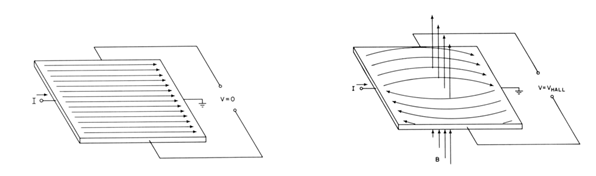Fig. 8: A Diagram Explaining the Half Effect Principle

The Hall voltage is a low level signal of the order of 20 to 30 microvolt’s in a magnetic field of one gauss. A signal of this magnitude requires a low noise, high impedance, moderate gain amplifier.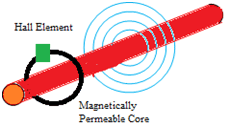Fig. 9: A Diagram Explaining the Half Voltage as a Low-Level Signal in a Magnetic Field

Hall sensors are based on following technologies. They can be used for measurement of DC, AC and impulse currents, with galvanic isolation between primary and secondary circuits

¨      Open Loop Hall effect technology
Current sensors based on this technology are electronic transformers. Primary current Icreates magnetic flux and the hall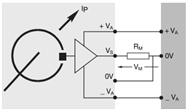Fig. 10: A Figure Explaining Current Sensors based on Open Loop Hall Effect Technology

probe placed in the airgap of the magnetic circuit provides a voltage proportional to the magnetic flux. This voltage itself is proportional to Ip is amplified and is used for further processing.

The linearity of the open-loop sensor is determined by the characteristics of the magnetic core and the Hall generator. Offset drift over temperature is determined primarily by the temperature sensitivity of the Hall generator.

¨      Closed Loop Hall effect technology
Current sensors based on this technology are also electronic transformers. Primary current Icreates magnetic flux and theFig. 11: A Figure Explaining Current Sensors based on Closed Loop Hall Effect Technology

hall probe placed in the airgap of the magnetic circuit provides a voltage proportional to the magnetic flux. This voltage is fed into a push-pull driver stage that drives the coil wound in series opposition on the magnetic core. Thus, it creates a magnetic field equal to and opposite to the field of the sensed current: maintaining the core flux level near zero. The secondary current cancels out the primary magnetic flux that created it (contra reaction). The output of the closed loop sensor is proportional to the aperture current and the number of turns of the coil.

Closed loop approach allows significant improvements in sensor performance by eliminating the influence of non-linearities in the magnetic core and by reducing the effects of temperature sensitivity in the Hall element

¨      Electronic technologyFig. 11: A figure Explaining Current Sensors based on Electronic Technology

In contrast to open loop and closed loop technology, they do not use magnetic circuit. Primary current Ip creates magnetic flux and different hall probes included in the sensor provides a voltage proportional to the magnetic flux.

Hall Effect based sensors do not suffer from Insertion loss (and related heating, etc.). However, frequency range, cost, DC offset, and external power represent the potential disadvantages of Hall-effect IC technology when compared to the resistive sensing methods.

·  Rogowski Coil
This device consists of a single-layer coil, uniformly wound on a non-magnetic core which is either flexible or formed into a circle which surrounds the conductor of the current to be measured. An AC current through the wire changes polarity. Changing polarity causes expanding and collapsing magnetic field which in turn induces current in the windings. The current is then processed to make suitable them suitable for measurement or control system.Fig. 12: A Diagram Demonstrating the Rogowski Coil

Practical implementations of this technique typically also incorporate a low-frequency roll-off to eliminate thermal noise and drift.The major benefit of a Rogowski Coil is that since the core is effectively air, there is no magnetic material to saturate and the coil's output remains linear for extremely high currents. This device is used for measuring high-energy current pulses or transients with high-frequency harmonic content since the upper bandwidth can extend into the megahertz range.

·  Transformer Technique
Transformer Technique is an extension of the Rogowski Coil technology wherein the air core is replaced with a material which concentrates the magnetic flux inside the coil. With the flux contained within the coil instead of passing through it, a direct relationship between the coil current and the current in the conductor generating the field is obtained.

Current sense transformers provide important benefits over simple resistive sensing. They offer electrical isolation, avoid insertion loss and they do not require external power. The lower power dissipation of a current sense transformer allows a much higher signal level, significantly improving the signal-to-noise environment of the control system.

Current transformers (CT) are commonly used in high-power systems to measure current. Major drawbacks are large size and cost and also the inability to detect DC Current.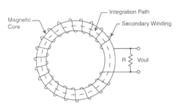Fig. 13: A diagram explaining use of current transformers in in high-power systems
·  Fiber optic current sensors
Development of magneto-optic sensors has demonstrated their use in current and magnetic field measurements applications. The principle of magneto-optic effects is based on the interaction between magnetic field and the phenomenon of light refraction and reflection in transparent medium and on its surface. They offer inherent immunity against EMI and good isolation against high voltages

Current sensors employ Faraday magneto-optic effect. Faraday effect causes the electromagnetic wave polarization rotation due to the magnetic field intensity in transparent material. The magnetic field induced by the current leads to an angle rotation in the plane of polarization of the linearly polarized light propagating across the ferromagnetic material. The rotation is detected by polarisers and analyzers at the input and output. By monitoring the rotation of incident polarization, magnetic field and hence current can be estimated.Fig. 14: A Diagram Demonstrating Fiber Optic Current Sensors

The magnitude of the effect depends further on the magnetooptic material constant (Verdet constant) and on the interaction length through which the wave travels in magnetized material.

## High Side v/s Low Side Sensing

SENSING TECHNIQUES: HIGH SIDE Vs LOW SIDE SENSING
There are two basic techniques for current sensing applications, viz., low side current sensing and high side current sensing, based upon the placement of sense resistor(between the supply and load or between load and ground).
·   Low-Side Current Sensing
Low-side current sensing connects the sensing resistor between the load and ground. Normally, the sensed voltage signal (VSEN = ISEN × RSEN) is so small that it needs to be amplified by subsequent op amp circuits to get the measurable output voltage (VOUT).Fig. 15: A Figure Illustrating Low-Side Current Sensing

¨      Low input Common mode voltage
¨      Ground referenced input and output
¨      Simplicity and low cost
¨      Ground path disturbance
¨      Load is lifted from system ground since RSEN adds undesirable resistance to the ground path.
¨      High load current caused by accidental short goes undetected
Low –side current sensing should be chosen when short circuit detection is not required and ground disturbances can be tolerated.

·  High-Side Current Sensing
High-side current sensing connects the sensing resistor between the power supply and load. The sensed voltage signal is amplified by subsequent op amp circuits to get the measurable VOUT.Fig. 16: A figure Illustrating High-Side Current Sensing

¨      Eliminates ground disturbance
¨      Load connects system ground directly
¨      Detects the high load current caused by accidental shorts
¨      Must be able to handle very high and dynamic Common mode input voltages
¨      Complexity and higher costs
High –side current sensing should be chosen when VCM range of the Difference amplifier is wide enough to withstand high Common mode input voltages

very nice

### hello fridets i just want

hello fridets

i just want know the how to interfacing current transformer in pic micro controller pls give the idea and give the circuit diagram  pls

regards

balaji

### thankyou so much

thankyou so much

### In my project iam using 400

In my project iam using 400/5A current transformer so which type of current sensor I have to select for that range and my project is to display the load.